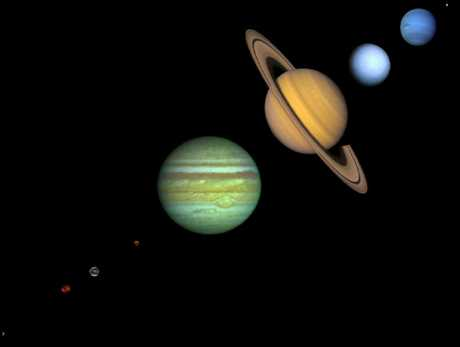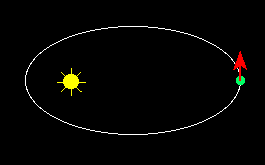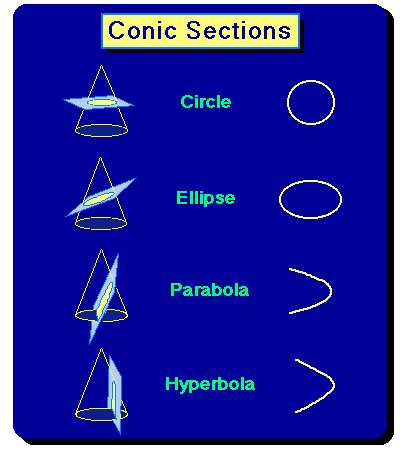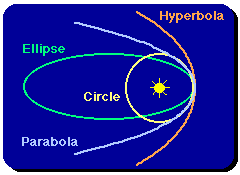Newtonian Gravitation and the
Laws of Kepler

We now come to the great synthesis of dynamics and astronomy accomplished by Newton: the Laws of Kepler for planetary motion may be derived from Newton's Law of Gravitation. Furthermore, Newton's Laws provide corrections to Kepler's Laws that turn out to be observable, and Newton's Law of Gravitation will be found to describe the motions of all objects in the heavens, not just the planets.

## Acceleration in Keplerian OrbitsKepler's Laws are illustrated in the adjacent animation. The red arrow indicates the instantaneous velocity vector at each point on the orbit (as always, we greatly exaggerate the eccentricty of the ellipse for purposes of illustration). Since the velocity is a vector, the direction of the velocity vector is indicated by the direction of the arrow and the magnitude of the velocity is indicated by the length of the arrow.

Notice that (because of Kepler's 2nd Law) the velocity vector is constantly changing both its magnitude and its direction as it moves around the elliptical orbit (if the orbit were circular, the magnitude of the velocity would remain constant but the direction would change continuously). Since either a change in the magnitude or the direction of the velocity vector constitutes an acceleration, there is a continuous acceleration as the planet moves about its orbit (whether circular or elliptical), and therefore by Newton's 2nd Law there is a force that acts at every point on the orbit. Furthermore, the force is not constant in magnitude, since the change in velocity (acceleration) is larger when the planet is near the Sun on the elliptical orbit.

## Newton's Laws and Kepler's Laws

Since this is a survey course, we shall not cover all the mathematics, but we now outline how Kepler's Laws are implied by those of Newton, and use Newton's Laws to supply corrections to Kepler's Laws.
1. Since the planets move on ellipses (Kepler's 1st Law), they are continually accelerating, as we have noted above. As we have also noted above, this implies a force acting continuously on the planets.

2. Because the planet-Sun line sweeps out equal areas in equal times (Kepler's 2nd Law), it is possible to show that the force must be directed toward the Sun from the planet.

3. From Kepler's 1st Law the orbit is an ellipse with the Sun at one focus; from Newton's laws it can be shown that this means that the magnitude of the force must vary as one over the square of the distance between the planet and the Sun.

4. Kepler's 3rd Law and Newton's 3rd Law imply that the force must be proportional to the product of the masses for the planet and the Sun.
Thus, Kepler's laws and Newton's laws taken together imply that the force that holds the planets in their orbits by continuously changing the planet's velocity so that it follows an elliptical path is (1) directed toward the Sun from the planet, (2) is proportional to the product of masses for the Sun and planet, and (3) is inversely proportional to the square of the planet-Sun separation. This is precisely the form of the gravitational force, with the universal gravitational constant G as the constant of proportionality. Thus, Newton's laws of motion, with a gravitational force used in the 2nd Law, imply Kepler's Laws, and the planets obey the same laws of motion as objects on the surface of the Earth!

## Conic Sections and Gravitational Orbits

As mentioned the ellipse is not the only possible orbit in a gravitational field. According to Newton's analysis, the possible orbits in a gravitational field can take the shape of the figures that are known as conic sections (so called because they may be obtained by slicing sections from a cone, as illustrated in the following figure).For the ellipse (and its special case, the circle), the plane intersects opposite "edges" of the cone. For the parabola the plane is parallel to one edge of the cone; for the hyperbola the plane is not parallel to an edge but it does not intersect opposite "edges" of the cone. (Remember that these cones extend forever downward; we have shown them with bottoms because we are only displaying a portion of the cone.)

## Examples of Gravitational Orbits

We see examples of all these possible orbitals in gravitational fields. In each case, the determining factor influencing the nature of the orbit is the relative speed of the object in its orbit as discussed above.• The orbits of some of the planets (e.g., Venus) are ellipses of such small eccentricity that they are essentially circles, and we can put artificial satellites into orbit around the Earth with circular orbits if we choose.

• The orbits of the planets generally are ellipses.

• Some comets have parabolic orbits; this means that they pass the Sun once and then leave the Solar System, never to return. Other comets have elliptical orbits and thus orbit the Sun with specific periods.

• The gravitational interaction between two passing stars generally results in hyperbolic trajectories for the two stars.
Thus, Kepler's elliptical orbitals are but one example of the possible orbits in a gravitational field. Only ellipses (and their special case, the circle) lead to bound orbits; the others are associated with one-time gravitational encounters. Here is a set of Java applets, taken from the Famous Curves Applet Index that illustrate the geometrical properties of these gravitational orbits:

• Java applet illustrating properties of a circle
• Java applet illustrating properties of an ellipse
• Java applet illustrating properties of an hyperbola
• Java applet illustrating properties of a parabola
For a given central force, increasing the velocity causes the orbit to change from a circle to an ellipse to a parabola to a hyperbola, with the changes occurring at certain critical velocities. For example, if the speed of the Earth (which is in a nearly circular gravitational orbit) were increased by about a factor of 1.4, the orbit would change into a parabola and the Earth would leave the Solar System.